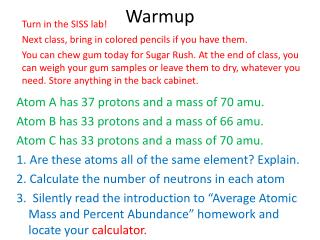DownloadDownload PresentationWarmup

# Warmup

Download Presentation## Warmup

- - - - - - - - - - - - - - - - - - - - - - - - - - - E N D - - - - - - - - - - - - - - - - - - - - - - - - - - -
##### Presentation Transcript

1. Warmup Turn in the SISS lab! Next class, bring in colored pencils if you have them. You can chew gum today for Sugar Rush. At the end of class, you can weigh your gum samples or leave them to dry, whatever you need. Store anything in the back cabinet. Atom A has 37 protons and a mass of 70 amu. Atom B has 33 protons and a mass of 66 amu. Atom C has 33 protons and a mass of 70 amu. 1. Are these atoms all of the same element? Explain. 2. Calculate the number of neutrons in each atom 3. Silently read the introduction to “Average Atomic Mass and Percent Abundance” homework and locate your calculator.

2. Average Atomic Mass

3. 1. The atomic mass of copper 63, is 62.93 amu, and has an abundance of 69.09%. Approximately 30.91% of copper atoms exist as copper 65, having an atomic mass of 64.9278 amu. The atomic mass shown on the periodic table for copper is 63.55 amu. How did scientists arrive at this value? % abundance of isotope 1 (in decimal form) average atomic mass for an element % abundance of isotope 2 (in decimal form) x atomic mass of isotope 1 + atomic mass of isotope 2 = x average atomic mass for Cu = 0.6909 x 62.93 + 0.3091 64.9278 x average atomic mass for Cu 43.478337 + 20.06918298 = a.a.mCu = 63.55 amu

4. 2. Naturally occurring sulfur consists of four isotopes, S-32 (95.0%), S-33 (0.76%), S-34 (4.22%), and S-36 (0.014%). Using these data, calculate the atomic weight of naturally occurring sulfur. The masses of the isotopes are: S-32: 31.97amu S-33: 32.97amu S-34: 33.97amu S-36: 35.97amu % abund. S-32 % abund. S-33 31.97 amu % abund. S-34 % abund. S-36 32.97 amu 33.97 amu x + 35.97 amu = x + x + x 31.97 amu 33.97 amu 35.97 amu 0.950 x 32.97 amu + 0.0076 0.0422 0.00014 = x + x + x 30.3715 + 0.250572 1.433534 0.0050358 = + + 32.06 amu (very close to the PT value)

5. 3. Rubidium has only 2 known stable isotopes. Calculate the % abundance of each isotope 85Rb = 84.9118 amu 87Rb = 86.9092 amu % abundance of isotope 1 (in decimal form) % abundance of isotope 2 (in decimal form) x atomic mass of isotope 1 + average atomic mass of Rb atomic mass of isotope 2 x = x x 84.9118 amu + y 85.47 amu 86.9092 amu x = y = 1 - x x 84.9118 amu 86.9092 amu 85.47 amu x + 1 - x x = 84.9118x + 1(86.9092) – x(86.9092) = 85.47 • 84.9118x + 86.9092 – 86.9092x = 85.47 • -1.9974 x = -1.4392 • -1.9974 -1.9974 x = 0.721, y = 0.279